# 如何模拟三维旋转机械

2015年 4月 30日

### 旋转机械的几何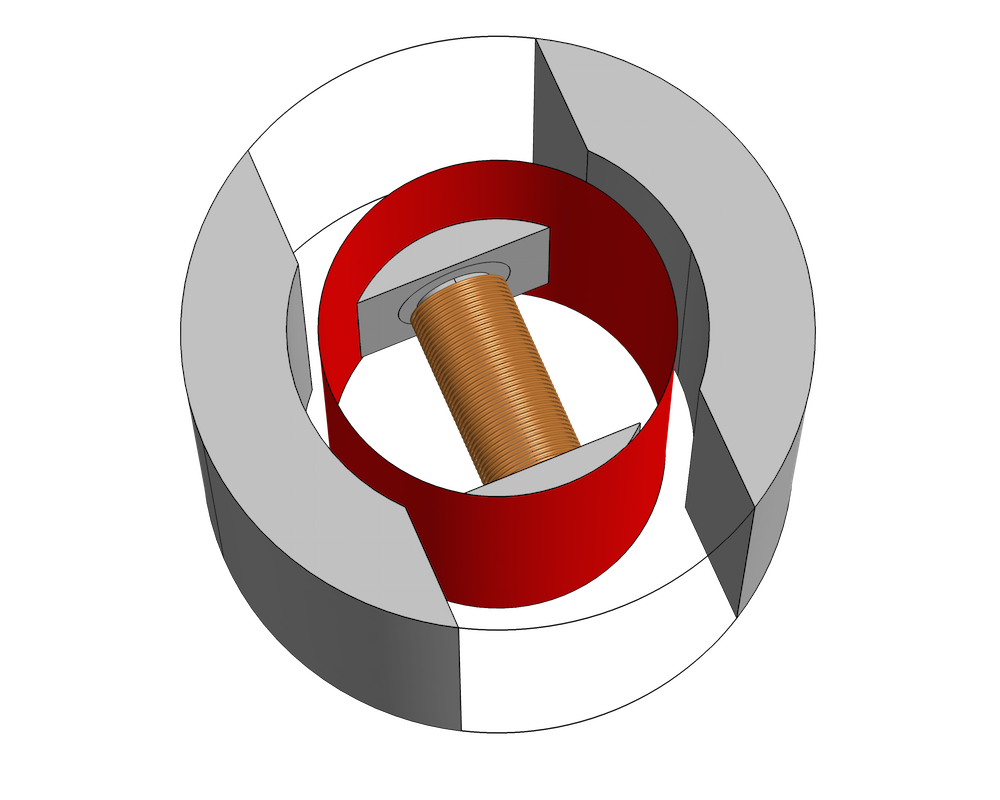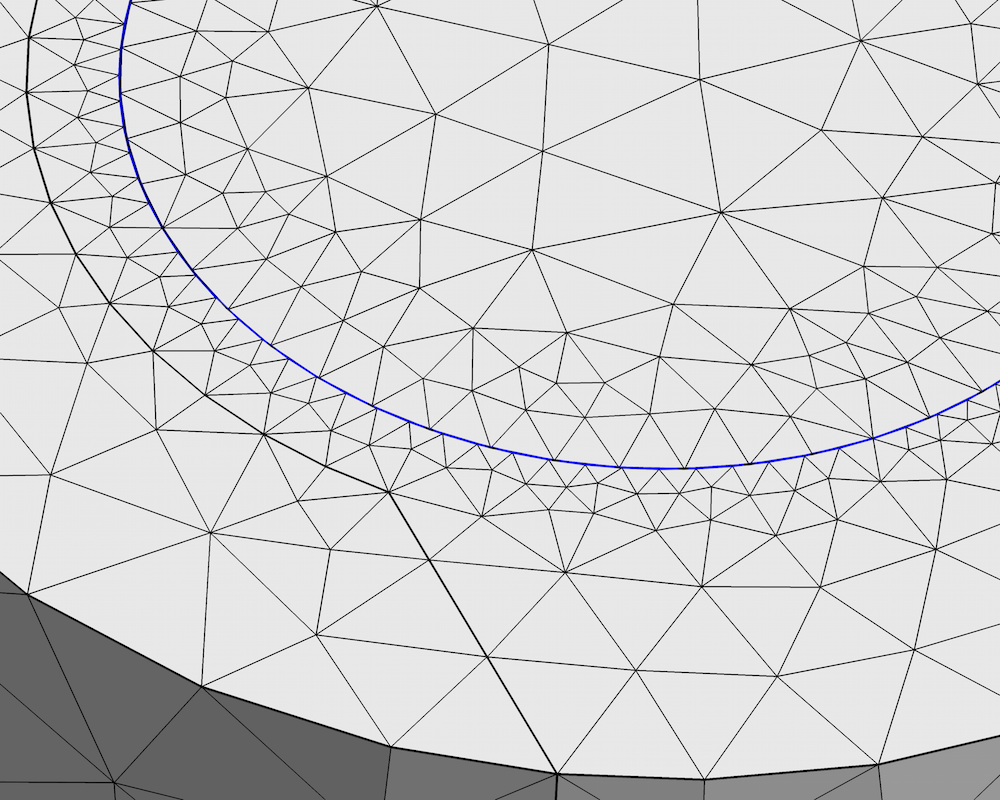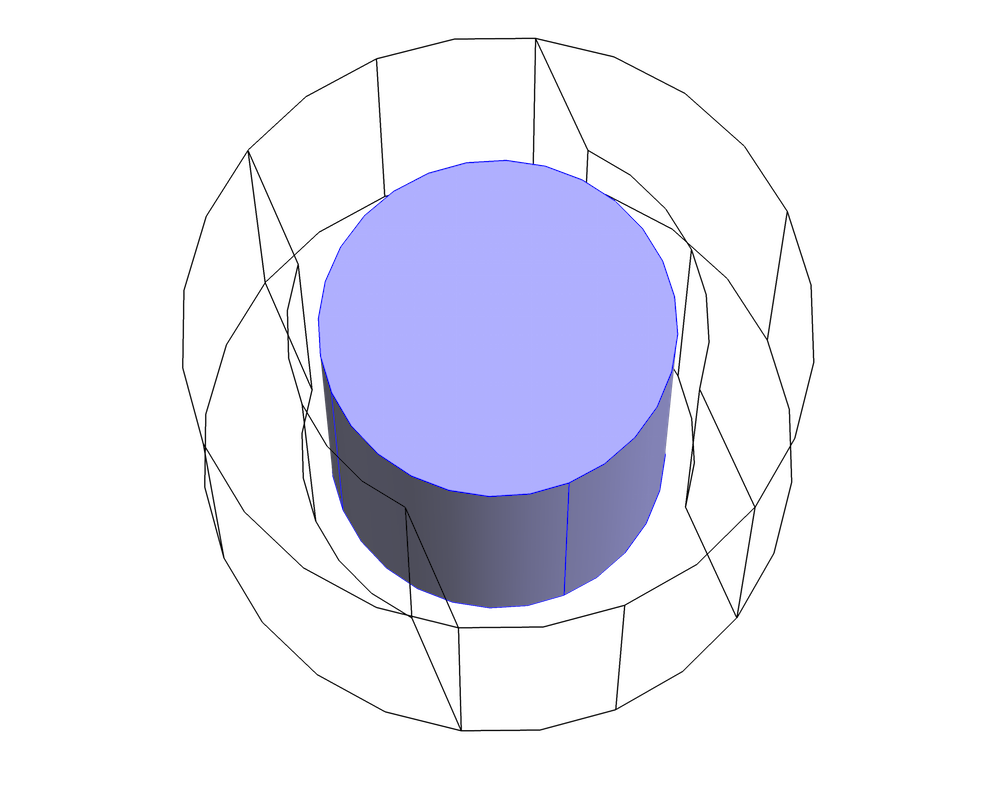“指定旋转”特征或“指定旋转速度”特征必须应用到包含转子的旋转部分。

### 混合公式

\begin{align}
\mathbf{E} &=-\frac{\partial \mathbf{A}}{\partial t} \\
\mathbf{B} &= \nabla \times \mathbf{A}
\end{align}

\begin{align}
\nabla \times \mathbf{E} &=-\frac{\partial \mathbf{B}}{\partial t} \\
\nabla \cdot \mathbf{B} &= 0
\end{align}

\nabla \times \mathbf{H} = \mathbf{J}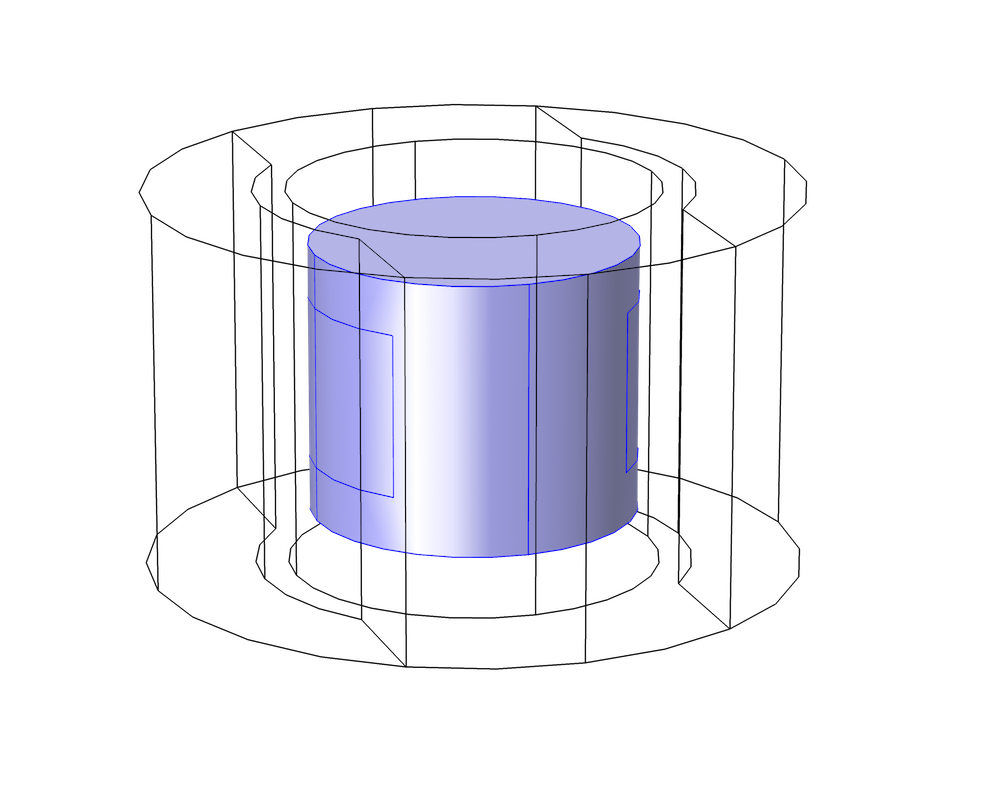“安培定律”特征仅应用于包含导电绕组的旋转部分的内部。注意，选定区域小于整个旋转部分，旋转部分延伸到切割边界。要提高精度，在一致对条件附近使用标量势公式。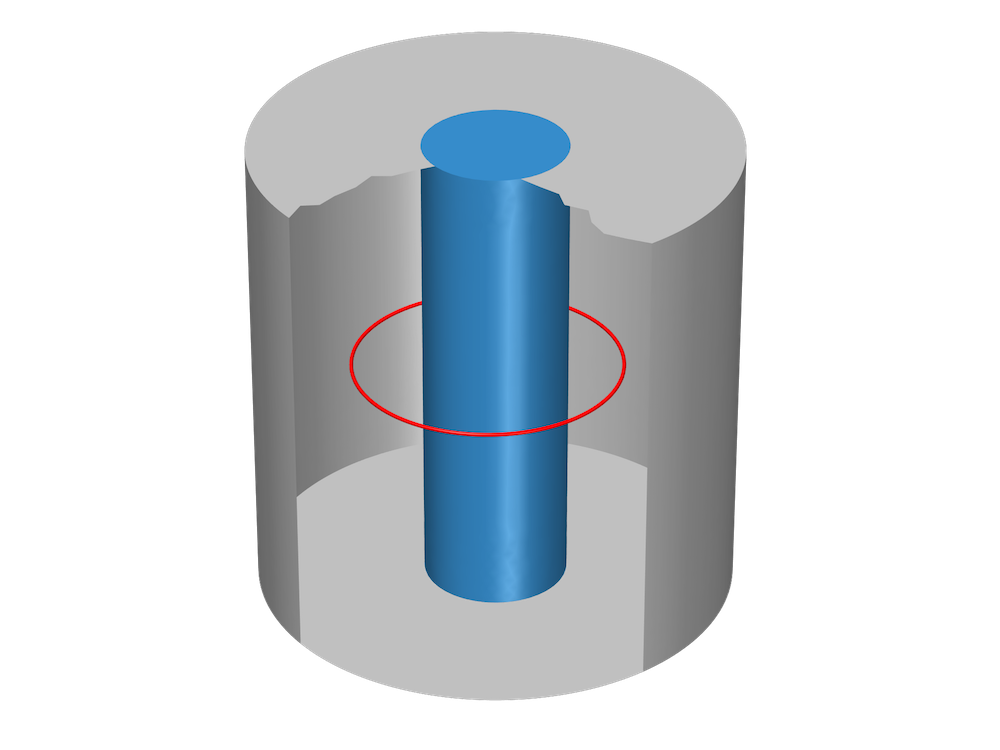### 相对运动和框架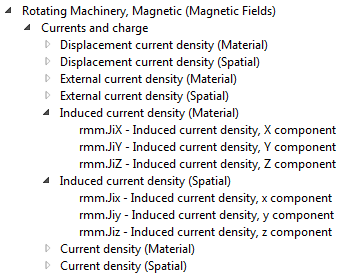\begin{align}
\mathbf{B}_\textrm{material} &= \mathbf{B}_\textrm{spatial} \\
\mathbf{E}_\textrm{material} &= \mathbf{E}_\textrm{spatial} + \mathbf{v} \times \mathbf{B}_\textrm{spatial}
\end{align}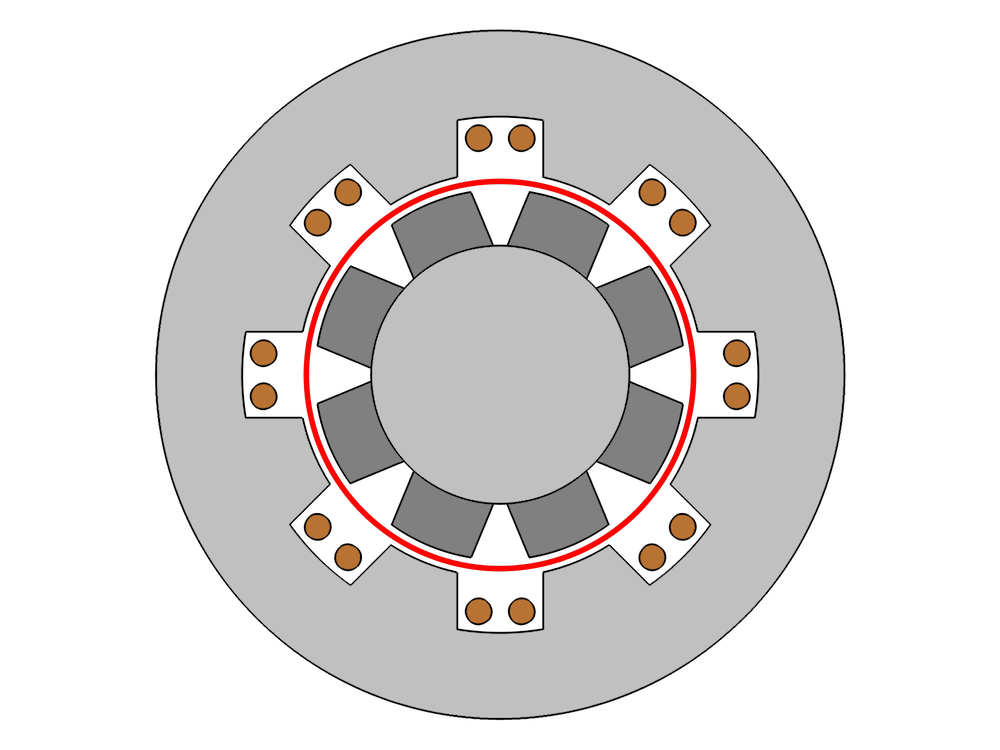### 延伸步骤

• 下载这些教程模型，自己动手尝试：